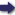(London :  Kegan Paul, Trench, Trübner & Co.,  1910.)

 Tools

## Search this bookPrev Page 35 Next``` CHAPTER LIT 35 The rationale of this calculation is the following:—If Explication we multiply, as we have done, by the number of the mentioned universal adhimdsa months, and divide the product by the universal solar months, the quotient represents the portion of adhimdsa time by which we have multiplied. As, now, the lunar months are the sum of solar and adhimdsa months, we multiply by them (the lunar months) and the division remains the same. The quo¬ tient is the sum of that number which is multiplied and that one which is sought for, i.e. the lunar days. We have already mentioned in the preceding part that by multiplying the lunar days by the universal Page 220. Unardtra days, and by dividing the product by the universal lunar days, we get the portion of unardtra days which belongs to the number of lunar days in question. However, the civil days in a kalpa are less than the lunar days by the amount of the Unardtra days. Now the lunar days we have stand in the same relation to the lunar days minus their due portion of unardtra days as the whole number of lunar days (of a kalpa) to the whole number of lunar days (of a kalpa) minus the complete number of unctrdira days (of a kalpa)', and the latter number are the universal civil days. If we, therefore, multiply the number of lunar days we have by the universal civil days, and divide the product by the universal lunar days, we get as quotient the number of civil days of the date in ques¬ tion, and that it was which we wanted to find. In- stead of multiplying by the whole sum of civil days (of a kalpa), we multiply by 3,506,481, and instead of dividing by the whole number of lunar days (of a kalpa), we divide by 3,562,220. The Hindus have still another method of calculation. Another It is the following:—" They multiply the elapsed years ahargana ot of the kalpa by 12, and add to the product the com¬ plete months which have elapsed of the current year. The sum they write down above the number 69,120, ```Prev Page 35 Next